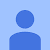# Amazon Fine Food Reviews : Case Study from Scratch

Source: Deep Learning on Medium

## Predicting the Sentiment of users whether the review given by user is positive or negative.Fig-1

### Objective

Given a review , we have to determine whether the review is positive (4 or 5) or negative(1 or 2).You can apply different models of machine learning and can see the best result out of different classification models to classify the reviews.

### Dataset

The dataset is available on the kaggle https://www.kaggle.com/snap/amazon-fine-food-reviews/home. This dataset consists of 568,454 reviews of fine food from Amazon.This dataset includes the reviews from Oct 1999 to Oct 2012.The dataset is avaliable in two forms

1. .csv file
2. .SQLite Database

### Importing the data

You can import the data which is of the form sqlite and you will not take the reviews of score 3(because it is hard to interpret as positive or negative).Now you will convert the score which are greater than 3 as “Positive” and score less than 3 as “Negative” . This conversion enable you to classify the review into binary classes(Positive or Negative).

`con = sqlite3.connect("./amazon-fine-food-reviews/database.sqlite")data = pd.read_sql_query('''SELECT *FROM REVIEWSWHERE SCORE != 3''', con)`
`data['Score'] = data["Score"].apply(lambda x: "positive" if x > 3 else "negative")`

### Data Cleaning

This stage is very important and quite time taking stage. You have to understand own your own what cleaning , you should do in our dataset and what type of cleaning is required according to our problem.Here, you will observe the duplicates in this dataset and you need to remove those duplicates in order to get the unbiased result for the analysis of data.One more important thing we need to focus on is that this dataset is based on timestamp so we need to sort our data according to the timestamp so that our model could perform very well on future unseen data (new reviews), for example, to deploy sentiment analysis of reviews in the system , each day there will be new reviews added for the different products which will act as new data for the system’s model so in order to perform well on new data with same results as it is preforming on old data, you need to sort them acc. to the timestamp.

`data = data[data.HelpfulnessNumerator <= data.HelpfulnessDenominator]`
`filtered_data = data.drop_duplicates(subset = {'UserId','ProfileName','Time'} ,keep = 'first', inplace = False)`
`final = filtered_data.sort_values('Time',axis= 0,inplace = False , na_position = 'last',ascending = True)`

### Text Preprocessing

Data preprocessing is also an important process in which you transform our data before applying the different models on dataset so that you can achieve the better results. In this stage , you can perform certain steps on our reviews(text) as mentioned below:-

• Begin with defining your own function which will remove the html tags or we can use Beautiful Soup .
• Removal of punctuations or limited set of special characters like . or “,” or removing whitespaces or etc from the reviews.
• Check if the word is made up of english letters and is not alpha-numeric.
• Check if the length of word is greater than 2 (as there is no adjective in 2 letters).
• uppercase to lower case conversion.
• Removal of Stopwords(commonly used word such as “a”, “the” etc to be ignored).
• Stemming(Snowball Stemming) the word of each reviews.

Code for cleaning html tags and punctuations in the reviews:

`def cleanhtml(sent):# function for cleaning html tags    cleanr = re.compile('<.*?>')    cleaned = re.sub(cleanr,' ',sent)    return cleaned`
`def cleanpunc(sent):# function for cleaning punctuations    clean = re.sub(r'[?|!|\$|#|\'|"|:]',r'',sent)    clean = re.sub(r'[,|(|)|.|\|/]',r' ',clean)    return clean`

Implementation:

`i=0all_positive_reviews =[]all_negative_reviews = []final_string = []stem_data = " "for p in final['Text'].values:    filtered_sens = []#filtered word    p = cleanhtml(p)    for w in p.split():       # print(w)        punc = cleanpunc(w)        for s in punc.split():            #print(w)            if (s.isalpha()) & (len(s)>2):                if s.lower() not in stop:                    stem_data = (st.stem(s.lower())).encode('utf8')                    filtered_sens.append(stem_data)                    if (final['Score'].values)[i] == 'positive':                        all_positive_reviews.append(stem_data)                    if (final['Score'].values)[i] == 'negative':                        all_negative_reviews.append(stem_data)                else:                    continue            else:                continue    #print(filtered_sens)    str1 = b" ".join(filtered_sens)    #print(str1)    final_string.append(str1)    i+=1`

### Featurization Techiniques on Text

To apply the different models on reviews , you have to convert text(reviews) to numerical vectors so that you can perform mathematical operations on it and finding the hyperplane which seperates the reviews into positive and negative. There are several featurization techniques some of them are discussed below:

• Bag Of Word

Theoritically :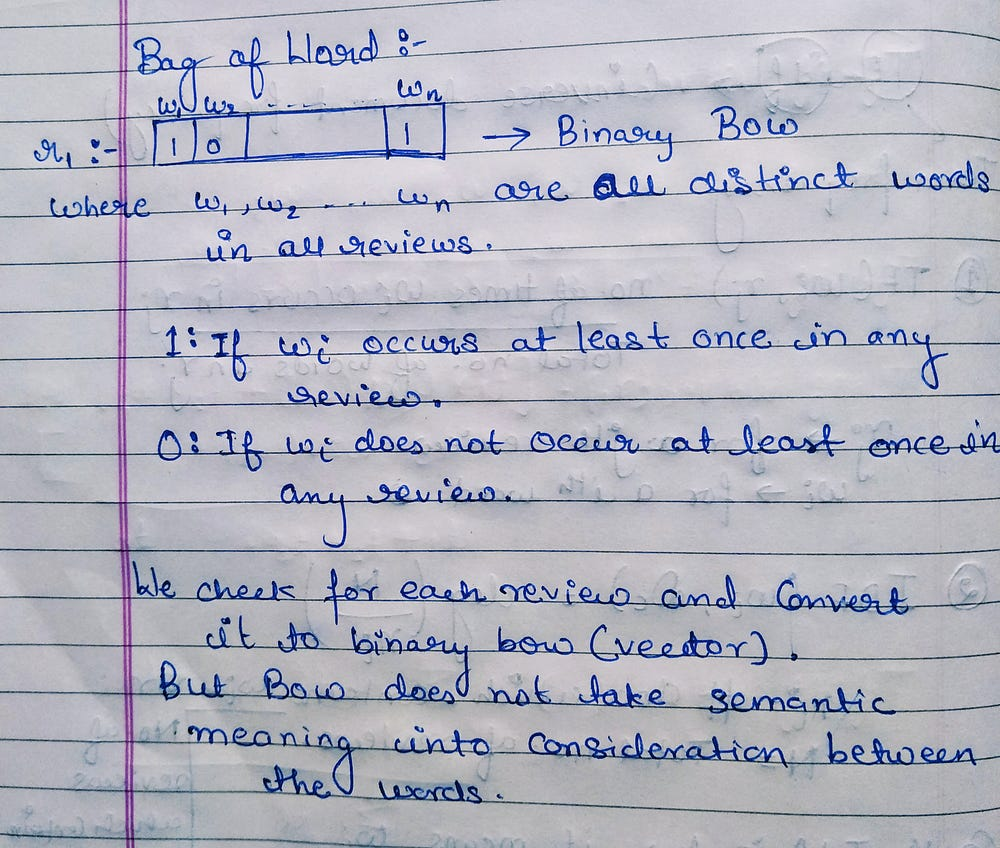Fig-2

Implementation:

`count_vect = CountVectorizer() #in scikit-learnbow_train = count_vect.fit_transform(X_train)bow_test = count_vect.transform(X_test)`
• Tf-idf

Theoritically: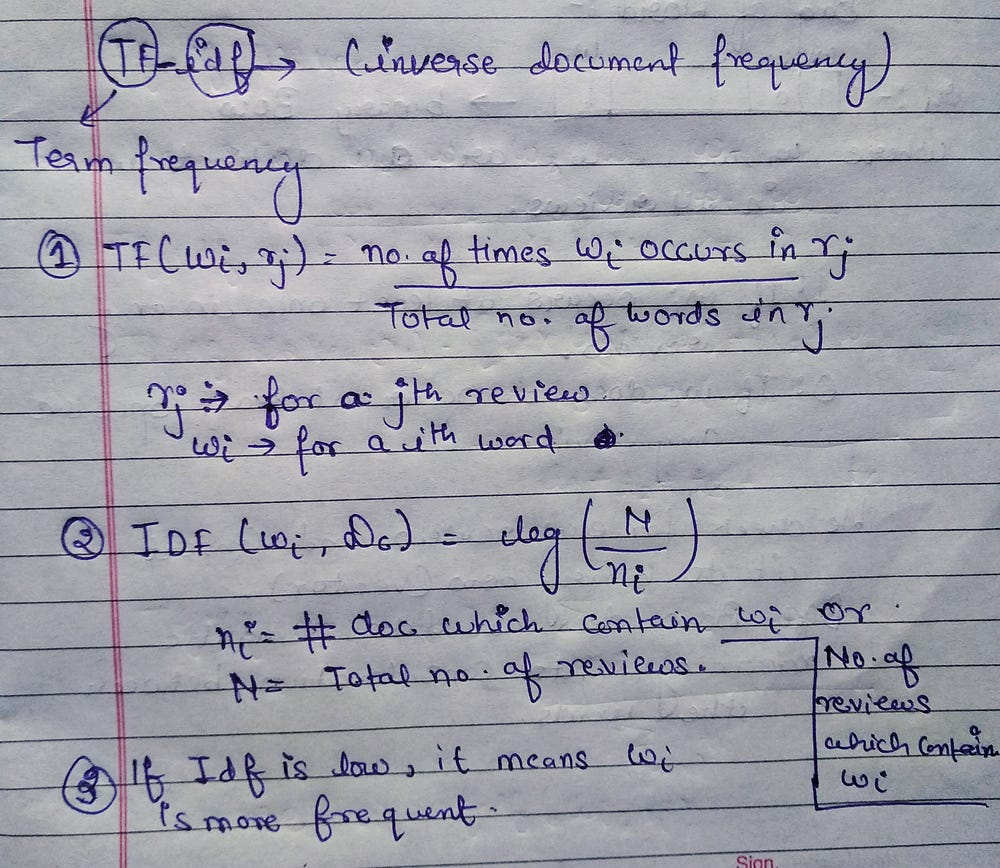Fig-3

Implementation:

`tfidf_vect = TfidfVectorizer()#in sklearntfidf_train = tfidf_vect.fit_transform (X_train)tfidf_test = tfidf_vect.transform(X_test)`
• Avg-w2vec

Theoritically: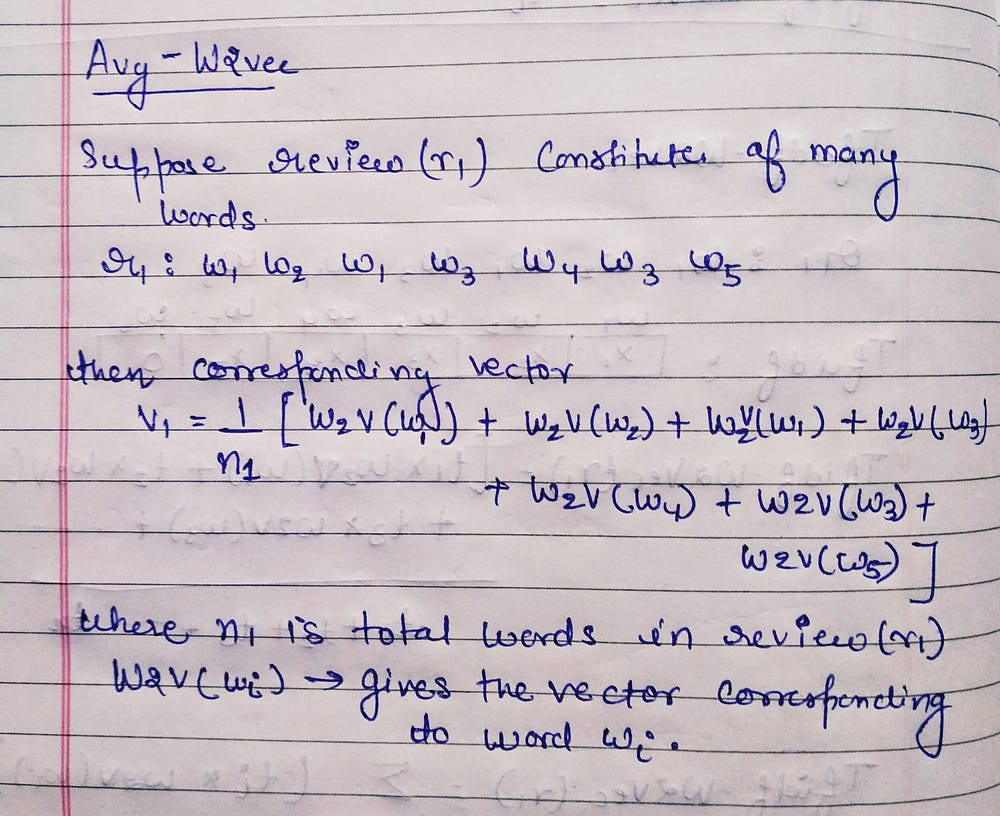Fig-4

Implementation:

`list_of_sent_train = []for i in X_train:    sent = []    for word in i.split():        sent.append(word.decode('utf-8'))    list_of_sent_train.append(sent)`
`from gensim.models import Word2Vecw2v_model = Word2Vec(list_of_sent_train,min_count = 5,size = 50,workers = 4)sent_vectors_train = []for sent in list_of_sent_train:    sent_vec = np.zeros(50)    cnt_word = 0    for word in sent:        try:            vec = w2v_model.wv[word]            sent_vec += vec            cnt_word += 1        except:            pass    sent_vec /= cnt_word    sent_vectors_train.append(sent_vec)print(len(sent_vectors_train))`
• Tfidf-w2vec

Theoritically: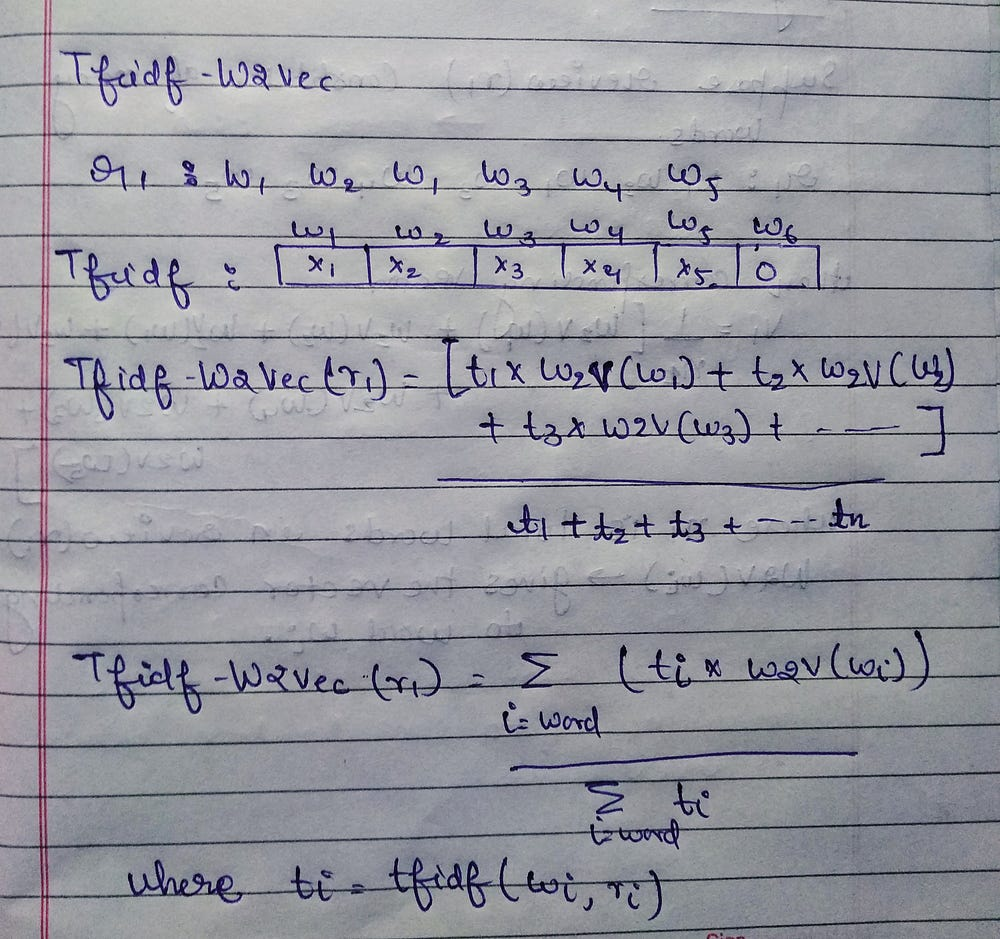Fig-5

Implementation:

`tf_idf_feat = tfidf_vect.get_feature_names()tfidf_sent_vec_train = []row = 0for sent in list_of_sent_train:    sent_vec = np.zeros(50)    weight_sum = 0    for word in sent:        try:            vec = w2v_model.wv[word]            tfidf = tfidf_train[row,tf_idf_feat.index(word)]            sent_vec += (vec*tfidf)            weight_sum += tfidf        except:            pass    sent_vec/= weight_sum    tfidf_sent_vec_train.append(sent_vec)    row += 1`

### Model as Logistic Regression

Logistic Regression as a classification model performed the best result in classifying the reviews as compared to other classification models. Logistic Regression is able to find the best hyperplane which could sepearte the reviews into positive and negative.

LogisticRegression(penalty=’l2’, dual=False, tol=0.0001, C=1.0, fit_intercept=True, intercept_scaling=1, class_weight=None, random_state=None, solver=’warn’, max_iter=100, multi_class=’warn’, verbose=0, warm_start=False, n_jobs=None)

Implementation on tfidf:

`param = [{'C':[10**i for i in range(-3,4)]}]model  = GridSearchCV(LogisticRegression(class_weight = 'balanced'),tunned_param , scoring = 'accuracy',cv = 10,n_jobs = -1)model.fit(X_tr,y_train)pred = model.predict(X_te)`

I have also applied different models on all of the featurization techniques and can check for the best result but i have found that logistic regression on tfidf performed the best result.

### Metrics For Evaluation:

You can use many metrics to determine your best model but choosing right metrics is again problem specific or problem dependent e.g in case of cancer diagnosis problem , we typically evaluate our model on basis of confusion metric(TN or TP) , etc not accuracy as only metrics. In this case study , i have evaluated model on basis of confusion metric(TN or TP), roc_auc_score , precision, recall, accuracy. Metrics for evaluating logistic regression on tfidf are mentioned below:

• Accuracy : 89.53
• Precision : 0.96(positive) and 0.56(negative)
• Recall : 0.91(positive) and 0.78(negative)
• AUC : 0.93

You can also check my work and can see the whole implementation here.

### Try it yourself

I hope you enjoyed this article , this case study will definitely improve your skills of machine learning on text data!.Feel free to leave suggestions or questions.